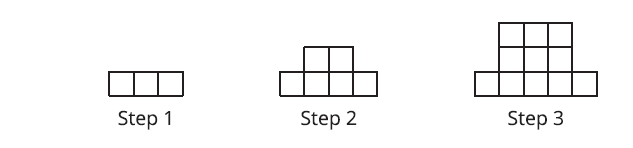# A.6.2.3 Expressing a Growth Pattern

Here is a pattern of squares.Complete the table below. Is the number of small squares growing linearly? Explain how you know.

The expression n² + 2 is called quadratic. The relationship between the step number and the number of squares is a quadratic relationship, which includes one quantity (the step number, in this case) being multiplied by itself to obtain a second quantity (the number of squares). In middle school, you expressed area of squares with side lengths s as s². The relationship between the side length and the area of the square is a quadratic relationship. s² is an example of a quadratic expression. The expression we wrote in this activity, is also an example of a quadratic expression. A quadratic expression can be written using a squared term, but it can be written in other ways as well. A quadratic relationship is not like a linear relationship because as one quantity increases by a certain amount, the second quantity doesn’t increase by the same amount.